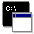## Firefly and PC GAMESS-related discussion club

Learn how to ask questions correctlySCF Convergence Problem during the Morokuma Decomposition Analysis

manoj
chem.manoj@gmail.com

Dear Sir/Ma'am
I was trying to apply Morokuma Decomposition analysis onto one of my H-bonded transition metal complex which is a dimer. Unfortunately the SCF is not converging. The input file is attached.

\$CONTRL SCFTYP=RHF RUNTYP=MOROKUMA MAXIT=400 ICHARG=0
IREST=-1 MULT=1  UNITS=angstrom EXETYP=RUN
INTTYP=HONDO ICUT=11 \$END
\$system TIMLIM=5970 MEMORY=50000000 \$end
\$smp csmtx=.t. call64=1 \$end
\$p2p p2p=.t. dlb=.t. \$end
\$BASIS GBASIS=N31 NGAUSS=6 NDFUNC=1 NPFUNC=1 \$END
\$GUESS GUESS=HUCKEL \$END
\$SCF NCONV=6 maxdii=30 \$END
\$MOROKM MOROKM=.TRUE. BSSE=.TRUE. IATM(1)=45 \$END
\$DATA
monomer corrin single point run
C1
....This message contains the 4 kb attachment [ Naked-H2O-US-Co-I-HF-EDA-2.inp ] This is the input file for Morokuma Energy Decomposition of a dimerSat Feb 18 '12 0:51amThis message read 1706 times